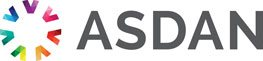# Lifeskills Challenge

## Working with decimals and percentage

### Challenge reference: 3110

Notional learning hours 20 1 The learner will develop skills and confidence in calculations involving decimals and percentage. Please log in to see the rest of this challenge

### Learning outcomes

What the learner needs to know, understand or be able to do

The learner will:

1. Know how to write, order and compare decimals and percentages.

2. Be able to perform simple calculations using decimals.

### Assessment criteria

What the learner need to demonstrate in order to meet the learning outcome

The learner can:

• Identify the value of a digit by its place in numbers with up to 3 decimal places
• Order and compare decimals and percentages by size
• Add and subtract numbers of up to 2 decimal places
• Multiply and divide numbers of up to 2 decimal places
• Multiply and divide decimals by 10 and 100
• Carry out rounding to a whole number and to 2 decimal places